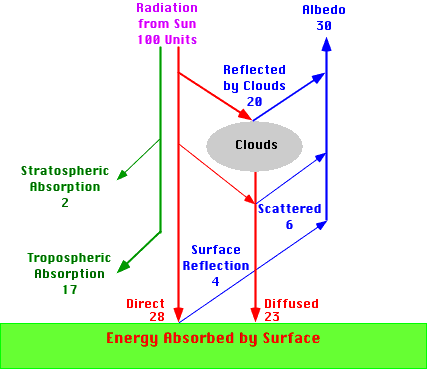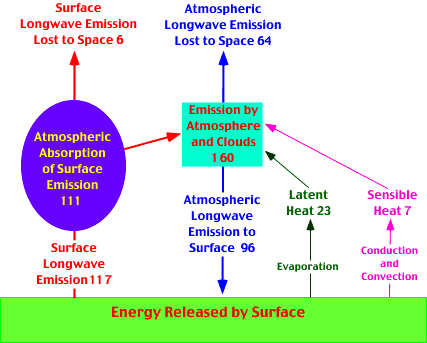PhysicalGeography.net | FUNDAMENTALS eBOOK

CHAPTER 7: Introduction to the Atmosphere

# (i). Net Radiation and the Planetary Energy Balance

Shortwave radiation from the Sun enters the surface-atmosphere system of the Earth and is ultimately returned to space as longwave radiation (because the Earth is cooler than the Sun). A basic necessity of this energy interchange is that incoming solar insolation and outgoing radiation be equal in quantity. One way of modeling this balance in energy exchange is described graphically with the use of the following two cascade diagrams.The Global Shortwave Radiation Cascade describes the relative amounts (based on 100 units available at the top of the atmosphere) of shortwave radiation partitioned to various atmospheric processes as it passes through the atmosphere. The diagram indicates that 19 units of insolation are absorbed (and therefore transferred into heat energy and longwave radiation) in the atmosphere by the following two processes:

Stratospheric Absorption of the Ultraviolet Radiation by Ozone 2 units; and

Tropospheric Absorption of Insolation by Clouds and Aerosols 17 units.

23 units of solar radiation are scattered in the atmosphere subsequently absorbed at the surface as diffused insolation. 28 units of the incoming solar radiation are absorbed at the surface as direct insolation. Total amount of solar insolation absorbed at the surface equals 51 units. The total amount of shortwave radiation absorbed at the surface and in the atmosphere is 70 units.

Three main losses of solar radiation back to space occur in the Earth's shortwave radiation cascade. 4 units of sunlight are returned to space from surface reflection. Cloud reflection returns another 20 units of solar radiation. Back scattering of sunlight returns 6 units to space. The total loss of shortwave radiation from these processes is 30 units. The term used to describe the combined effect of all of these shortwave losses is Earth albedo.The Global Longwave Radiation Cascade indicates that energy leaves the Earth's surface through three different processes. 7 units leave the surface as sensible heat. This heat is transferred into the atmosphere by conduction and convection. The melting and evaporation of water at the Earth's surface incorporates 23 units energy into the atmosphere as latent heat. This latent heat is released into the atmosphere when the water condenses or becomes solid. Both of these processes become part of the emission of longwave radiation by the atmosphere and clouds.

The surface of the Earth emits 117 units of longwave radiation. Of this emission only 6 units are directly lost to space. The other 111 units are absorbed by greenhouse gases in the atmosphere and converted into heat energy and then into atmospheric emissions of longwave radiation (the greenhouse effect).

The atmosphere emits 160 units of longwave energy. Contributions to this 160 units are from surface emissions of longwave radiation (111 units), latent heat transfer (23 units), sensible heat transfer (7 units), and the absorption of shortwave radiation by atmospheric gases and clouds (19 units, see Figure 7i-1) . Atmospheric emissions travel in two directions. 64 units of atmospheric emission is lost directly to space. 96 units travel to the Earth's surface where it is absorbed and transferred into heat energy.

The total amount of energy lost to space in the global longwave radiation cascade is 70 units (surface emission 6 units + atmospheric emission 64 units.) This is the same amount of energy that was added to the Earth's atmosphere and surface by the Global Shortwave Radiation Cascade.

Finally, to balance the surface energy exchanges in this cascade we have to account for 51 units of missing energy [atmosphere and cloud longwave emission (96 units) minus surface longwave emission (117 units) minus latent heat transfer (23 units) minus sensible heat transfer (7 units) = -51 units]. This missing component to the radiation balance is the 51 units of energy absorbed at the Earth's surface as direct and diffused shortwave radiation (see Figure 7i-1).

The following equations can be used to mathematically model net shortwave radiation balance, net longwave radiation balance, and net radiation balance for the Earth's surface at a single location or for the whole globe for any temporal period:

K* = (K + k)(1 - a)

L* = (LD - LU)

Q* = (K + k)(1 - a) -LU + LD

where

Q* is surface net radiation (global annual values of Q* = 0 , because input equals output, local values can be positive or negative),

K* is surface net shortwave radiation,

K is surface direct shortwave radiation,

k is diffused shortwave radiation (scattered insolation) at the surface,

a is the albedo of surface,

L* is net longwave radiation at the surface,

LD is atmospheric counter-radiation (see Greenhouse Effect) directed to the Earth's surface, and

LU is longwave radiation lost from the Earth's surface.

NASA's Surface Radiation Budget Project has used satellite data, computer models, and meteorological data to determine surface net shortwave radiation, net longwave radiation, and net radiation balances for the period July 1983 to June 1991. The following links display these balances for January and July globally:

Average Net Shortwave Radiation at the Earth's Surface: January 1984-1991 (K*)

Average Net Shortwave Radiation at the Earth's Surface: July 1983-1990 (K*)

Average Net Longwave Radiation at the Earth's Surface: January 1984-1991 (L*)

Average Net Longwave Radiation at the Earth's Surface: July 1983-1990 (L*)

Average Net Radiation at the Earth's Surface: January 1984-1991 (Q*)

Average Net Radiation at the Earth's Surface: July 1983-1990 (Q*)

Study Guide